# BUSDUCT SYSTEM DESIGN PART-4

In order to have low losses and enhanced reliability in the distribution system, bus-ducts are preferred over cables for higher current ratings and shorter lengths at different voltage levels. Though Sandwich Bustrunking System with rated operational voltage (Ue) 1000 Volt are easily available, when it comes to economics–Air insulated bus ducts have an edge. This article focuses on the types of air insulated busducts and their selection guidelines, design requirements, de-rating factors and sizing requirement...Busbar enclosure

The proximity effect can also be minimized by using a non-magnetic enclosure of aluminium or stainless steel. In magnetic materials the field in the enclosure is produced in the form of small magnetic loops. Its effect cannot be alleviated by breaking the electrical path alone, as illustrated in Figure-13. Its effect can be reduced only by replacing a few parts of the magnetic enclosure itself, such as its top or bottom covers or both, with a non-magnetic material. It is possible to achieve an economical and low-loss enclosure by replacing only its top and bottom covers with a non-magnetic material. The covers constitute the larger part of the surface area of the enclosure.

By equipping adequate louvers in the enclosure or by using a forced-air draught through the length of the enclosure.

Designing A 2500A Aluminium Busbar System

Example-6: For a three phase four wire 415V ±10%, 50Hz ±3% Fault level – 50kA, Duration of fault – 1sec, Continuous current rating – 2500A,

Ambient temperature – 50deg.C, with maximum permissible operating temperature – 85deg.C Permissible final temperature at the end of the fault – 185deg.C, sizing of busduct of different types are detailed below

Case-1: Non segregated phase busbar without phase interleaving

Minimum size of busbars for short circuit conditions:185-85 = (1.166/100)* (50000/A)2.(1+0.0040385)1 A = 625 mm2

Assume rectangular flats of electrolytic grade E-91E or its equivalent. Busbars chosen for each phase – four (152.4 mm x 6.35 mm) – which are more than the minimum size required to account for the thermal effects during a shortcircuit condition and for neutral – two (152.4 x 6.35 mm) Size of busbar enclosure – 870 mm x 380 mm (Figure-15) Material of enclosure – Aluminium Busbar configuration – as in Figure 14.

Deratings:

• Due to ambient temperature, from table-1

K1 = 0.815

• Due to size of enclosure

Heat dissipation factor = Cross sectional area of active Aluminium / Area of enclosure =

= 0.041 (4%)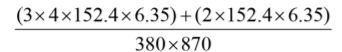From table-3, K2 = 0.77

• Due to proximity effect, as S < 2b, derating is approximately 20%

K3 = 0.8

Total derating K = 0.8150.770.8 = 0.5

Busbar current rating of 4×152.4mmx6.35mm Aluminium busbars per phase as in table-11 = 4240A. Effective rating after considering all de-ratings = 4240*0.5 = 2120A

These sections of busbars are not adequate for the required current rating of 2500A. The rating of the bus system can however be enhanced by almost 20% and make it suitable for the required rating by providing the busbars and inside of the enclosure with a non-metallic, matt finish black paint.

Voltage drop: Skin effect:

Rdc for E-91E grade of conductor from table-13

= 32.38 x 10-6 ohm per conductor at 200C

Rdc at an operating temperature of 850C

= Rdc20 (1+a20(q2-q1))

= 32.38 (1+0.00363 (85-20))

= 40.02 x 10-6 ohm per conductor and for the phase = 40.02 / 4 = 10.005 x 10-3 ohm/1000m

Area of cross section per phase = 4×152.4×6.35 = 38.71cm2. For this area of cross section, the skin effect ratio Rac/Rdc from fig-4 for Aluminium grade E-91E at 850C having b/a = 152.4/44.45 ≈ 4 measures almost 1.425 approx.

So Rdc/Rac = 1.425

® Rac = 10.005 x 10-3 x 1.425 = 14.257 x 10-3 ohm/1000m per phase

Proximity effect:

Measure reactance Xa from fig-9 for 1.26 x S/(a+b)

= 1.26*210/(44.45+152.4)

= 1.344 corresponding to a/b = 44.45/152.4 = 0.29

Xa = 110 x 10-6 ohm per meter per phase

Impedance Z == 0.111 ohm per 1000m per phase

For 20m length of busduct this impedance will cause a voltage drop of 2500×0.111×20/1000 = 5.5V which is 1.3% of rated voltage and is therefore acceptable.

Calculation of short circuit effects:

Electrodynamic forces:

Isc = 50,000AK corresponding to (S-a)/(a+b) i.e. (210-44.45)/(44.45+152.4) = 0.84 from curve for a/b = 0.25 is 0.93 approx.= 17,714.6 N/m = 1806.1 kg/m (1N/m = 1/9.807 kg/m) Since the busbar support are assumed to be at a distance of 400mm Force on each section of busbars, insulators and the mounting fasteners

= 1806.1×0.4 = 722.51kg

Mechanical suitability of busbars and their supporting system: Bending stress on the busbars Bending stress at section x-x =Where Fm = maximum electrodynamic forces acting on each support in the event of fault

I = Centre distance between two busbar supports

N = Number of busbars per phase

M = Sectional modulus at section x-x = (1/6)ab2 cm

Fm = 722.51 kg

I = 40cm

a = 6.35 mm = 0.635cm

b = 152.4 mm = 15.24 cm

N = 4

M = 0.63515.2415.24/6 = 24.58cm3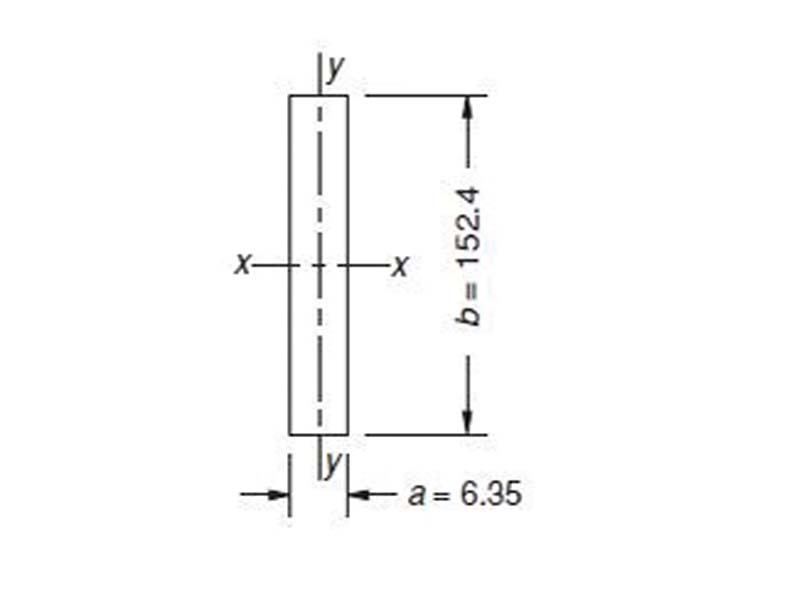Bending stress =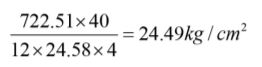From Table-13, Busbar support can be SMC or DMC

Case-2: Non segregated phase busbar with phase interleaving

Busbar current rating of 2×152.4mmx6.35mm Aluminium busbars per phase as in table-11 = 2860A

Total current = 2*2860 = 5720A Figure-16:

With derating = 0.5*5720 = 2860A

Voltage drop: Skin effect:

Rdc for E-91E grade of conductor from table-13
= 32.38 x 10-6 ohm per conductor at 200C

Rdc at an operating temperature of 850C

= Rdc20 (1+a20(q2-q1))

= 32.38 (1+0.00363 (85-20))

= 40.02 x 10-6 ohm per conductor and for the phase = 40.02 / 4 = 10.005 x 10-3 ohm/1000m

Area of cross section per phase = 4×152.4×6.35 = 38.71cm2 For this area of cross section, the skin effect ratio Rac/Rdc from fig-4 for Aluminium grade E-91E at 85 deg,C having b/a = 152.4/19.05 ≈ 8

® Rac = 10.005 x 10-3 x 1.18 = 11.81 x 10-3 ohm/1000m per phaseProximity effect:

Measure reactance Xa from fig-9 for 1.26 x S/(a+b) = 1.26*99.05/(19.05+152.4) = 0.73 corresponding to a/b = 19.05/152.4 = 0.125

Xa = 74 x 10-6 ohm per meter per circuit

Combined Xa = 37 x 10-6 ohm per meter

Impedance Z = 0.0388 ohm per 1000m per phase For 20m length of busduct this impedance will cause a voltage drop of 2500×0.038×20/1000 = 1.9V which is 0.45% of rated voltage and is therefore acceptable.Calculation of short circuit effects:

Electrodynamic forces: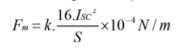Isc = 50,000A

K corresponding to (S-a)/(a+b) i.e. (99.05-19.05)/(19.05+152.4) = 0.47 from curve for a/b = 0.125 is 0.77 approx.= 15,547 N/m = 1585.3 kg/m (1N/m = 1/9.807 kg/m )

Since the busbar support are assumed to be at a distance of 400mm. Force on each section of busbars, insulators and the mounting fasteners

= 1585.3×0.4 = 634.2kg

Table-7: Properties of copper and Aluminium at 200C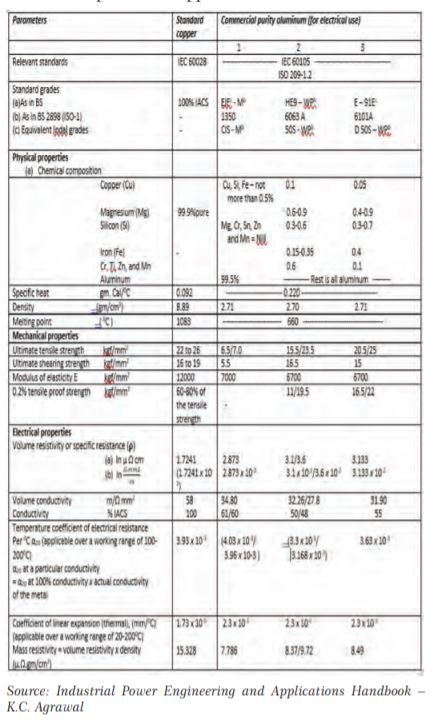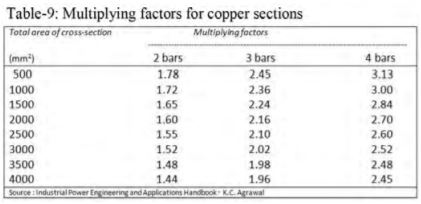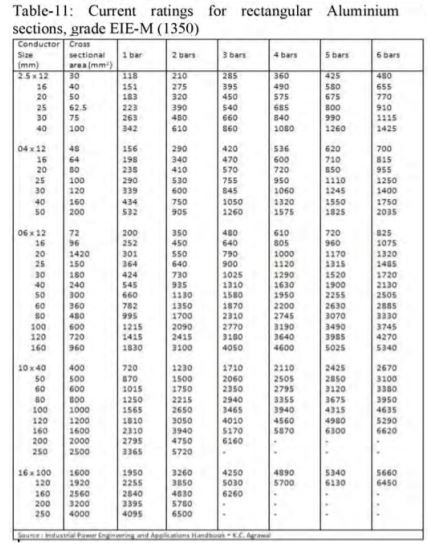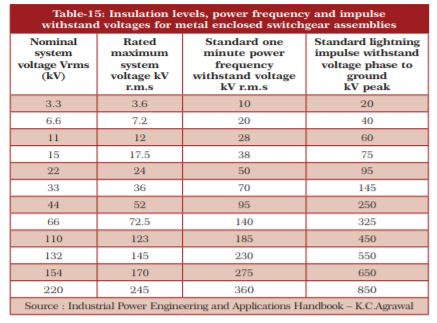Conclusion:

This article gave a comprehensive insight on air insulated busduct design and selection guidelines. Bus-duct design must be based on electromagnetic, thermal and mechanical considerations and all dominant factors upon these analyses were briefed in this paper.Chanukya Annepu

The author is from the Engineering Services Division, Indira Gandhi Centre for Atomic Research, Kalpakkam, India